Next: LSJIMP: Least-squares minimization Up: 2-D Theory \label>chapter:theory2d> Previous: 2-D Theory \label>chapter:theory2d>

# The LSJIMP Inverse problem

Figuremotivates LSJIMP. The Figure assumes that the recorded data is the superposition of primary events and pegleg multiples from the seabed. In effect, the images constructed from the primaries and from each mode of multiple constitute independent measurements of the earth's reflectivity at depth. Unfortunately, these independent measurements are embedded in a single data record. We would like to improve signal-to-noise ratio or fill illumination gaps by averaging the images. However, simple averaging of the raw images encounters two problems. First, unless the multiple images have undergone an appropriate amplitude correction, the signal events are incommensurable. Secondly, corresponding crosstalk events on the primary and multiple images are kinematically quite consistent, especially at near offsets, meaning that averaging the images will do little to increase the signal-to-noise ratio or signal fidelity.

The previous paragraph underscores the main obstacle facing algorithms which attempt to jointly image multiples and primaries: while multiples provide additional information about the earth's reflectivity, we cannot exploit it unless we separate the individual modes. Cleanly separating a variety of different multiple modes from prestack data is both expensive and difficult. Moreover, by casting mode separation as a preprocessing step, as is the norm, we risk biasing the amplitudes in the separated modes, which could inhibit the integration.

LSJIMP solves the separation and integration problems simultaneously, as a global least-squares inversion problem. The model space, as illustrated in Figure, contains a collection of images, with the energy from each mode partitioned into one, and only one image. Moreover, each image has a special form: because the forward modeling operator contains appropriate amplitude correction operators the signal events in multiple and primary images are directly comparable, in terms of both kinematics and amplitudes. In order to solve the integration problem, we can apply a model regularization operator which penalizes inconsistency between images, and thus spreads'' signal from one model panel to another, using the multiples to fill gaps in the primary illumination and to increase signal fidelity.

LSJIMP models the recorded data as the superposition of primary reflections and p orders of pegleg multiples from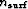multiple-generating surfaces. An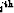order pegleg splits into i+1 legs. If we denote the primaries asand theleg of theorder pegleg from themultiple generator as, the modeled data takes the following form: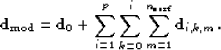(1)
Figureillustrates a simple case, where we model only first-order peglegs from a the seabed ().

Other authors have solved a similar least-squares problem. Nemeth et al. (1999) jointly imaged and separated compressional waves and various (non-multiple) embedded coherent noise modes. Guitton et al. (2001) used nonstationary prediction-error filters to model primaries and surface-related multiples, but cast the problem purely in terms of wavefield separation, rather than joint imaging of multiples and primaries.

If we have designed an imaging operator that produces primary and multiple images with consistent signal (kinematics and angle-dependent amplitudes), then we assume that we can model the important events in the data. Let us denote the modeling operator (adjoint to imaging) for primaries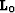and the image of the primaries. Similarly, for theleg of theorder pegleg from themultiple generator, we denote the modeling operator and imageand, respectively. Following Figure, we can rewrite equation ():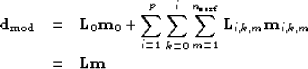(2) (3)